Next: 12. Power Series Up: 11. Infinite Series Previous: 11.3 Alternating Series   Index

# 11.4 Absolute Convergence

11.39   Definition (Absolute Convergence.) Let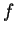be a complex sequence. We say thatis absolutely summable if and only if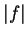is summable; i.e., if and only ifconverges. In this case, we also say that the series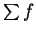is absolutely convergent.

11.40   Example.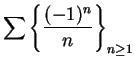is convergent, but is not absolutely convergent.

11.41   Theorem. Letbe a complex sequence. Ifis absolutely convergent, thenis convergent.

Proof:

Case 1:
Suppose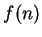is real for all, and that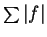converges. Then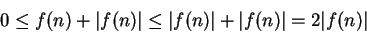for all, so by the comparison test,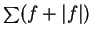converges. Then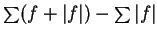, being the difference of two convergent sequences, is convergent; i.e.,converges.

Case 2:
Supposeis an arbitrary absolutely convergent complex series. We know that for all,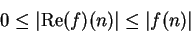and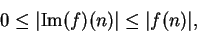so by the comparison test,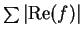and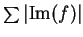are convergent, and by Case 1,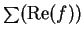and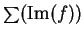are convergent. It follows thatis convergent.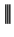11.42   Example. Let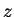be a non-zero complex number. Let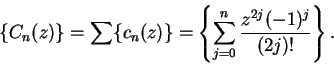I claim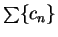is absolutely convergent (and hence convergent). We have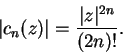We have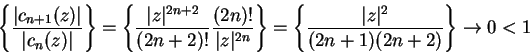so by the ratio test,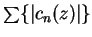converges. Hence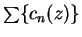is absolutely convergent, and hence it is convergent. Clearly, soconverges for all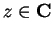. In the exercises you will show that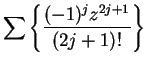is also convergent for all.

Motivated by the results of section 10.3, we make the following definitions:

11.43   Definition (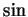and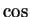.) For all, we define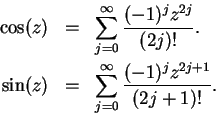11.44   Remark. It is clear from the definition thatFor all,, let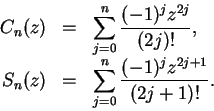Then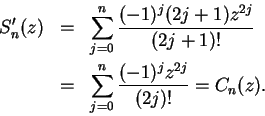I would now like to be able to say that for all,i.e., I would like to have a theorem that saysHowever, the next example shows that this hoped for theorem is not true.

11.45   Example. Let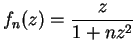for all,. Then for all,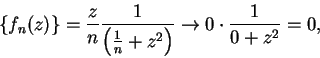and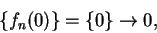so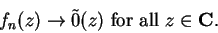Now. Sofor all, and thus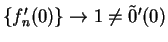. Eventually we will show thatand, but it will require some work.

11.46   Warning. Defining sine and cosine in terms of infinite series can be dangerous to the well being of the definer. In 1933 Edmund Landau was forced to resign from his position at the University of Göttingen as a result of a Nazi-organized boycott of his lectures. Among other things, it was claimed that Landau's definitions of sine and cosine in terms of power series was un-German'', and that the definitions lacked sense and meaning''[33, pp 226-227].

11.47   Exercise. Show that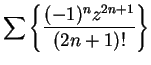converges for all.

11.48   Exercise. A
a) Does the series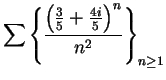converge?
b) Does the sequence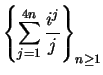converge?

11.49   Exercise. A
a) For what complex numbersdoes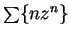converge?
b) For what complex numbersdoes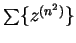converge?

11.50   Note. The harmonic series was shown to be unbounded by Nicole
Oresme c. 1360 [31, p437]. However, many 17th and 18th century mathematicians believed that (in our terminology) every null sequence is summable. Jacob Bernoulli rediscovered Oresme's result in 1687, and reported that it contradicted his earlier belief that an infinite series whose last term vanishes must be finite[31, p 437]. As late as 1770, Lagrange said that a series represents a number if itsth term approaches[31, p 464].

The ratio test was stated by Jean D'Alembert in 1768, and by Edward Waring in 1776[31, p 465]. D'Alembert knew that the ratio test guaranteed absolute convergence.

The alternating series test appears in a letter from Leibniz to Jacob Bernoulli written in 1713[31, p461].

The series (11.35) for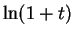is called Mercator's formula after Nicolaus Mercator who published it in 1668. It was discovered earlier by Newton in 1664 when he was an undergraduate at Cambridge. After Newton read Mercator's book, he quickly wrote down some of his own ideas (which were much more general than Mercator's) and allowed his notes to be circulated, but not published. Newton used the logarithm formula to calculate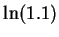to 68 decimals (of which the 28th and 43rd were wrong), but a few years later, he redid the calculation and corrected the errors.

See [22, chapter 2] for a discussion of Newton's work on series.

The series representation for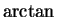(11.37) is called Gregory's formula after John Gregory (1638-1675) or Leibniz's formula after Gottfried Leibniz (1646-1716). However, it was known to sixteenth century Indian mathematicians who credited it to Madhava (c. 1340-1425). The Indian version was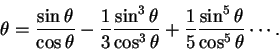(See[30, p292].)Next: 12. Power Series Up: 11. Infinite Series Previous: 11.3 Alternating Series   Index